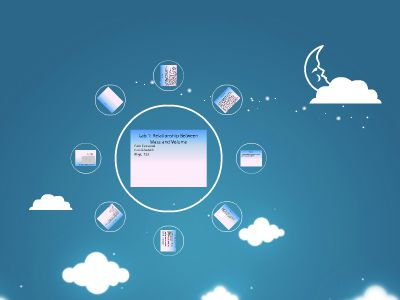Publications: 106 | Followers: 1

## Lab 1_ Relationship Between Mass and Volume

Publish on Category: Birds 0

Lab 1: Relationship Between Mass and Volume
Faith EastwoodNataliaSedykhPhys. 122
Purpose
What is the relationship between mass and volume for a copper cylinder.
Theory
There will be a direct linear relationship between volume and mass. As Volume increases mass will increase.
Materials and Procedures
Materials: Balance scale, solid copper cylinders, micrometerProcedures:Measure the mass using a balance scale.Measure the length and the diameter using the micrometerCalculate the volume using the formula (3.14r^2)(h)Repeat steps 1-3 with each cylinder
Uncertainty
Uncertainty:To determine the uncertainty of our measurement devices we took 10 readings with the balance scale and 10 readings with the micrometer.Uncertainty for length:Average 1.289 cmMax 1.230 cmMin 1.226 cmUncertainty .063 cmUncertainty for mass:Average 29.29 gMax 29.31 gMin 29.25 gUncertainty .04 g
Data and Sample Calculation
Sample calculation for volumeV=Πr^2*hV=3.14*.634cm^2*1.228cmV= 1.550 cm^3
Graph
Analysis
Fromour graph we can tell that there is a positive linear relationship between volume and mass. As the volume of our cylinders increased the mass increased as well.The equation for the line is y=8.97g/cm^3This means that for every increase in volume of 1 cm^3 the mass increased by 8.97 g.The units for our slope, g/cm^3) are also the units for density. Meaning that the sample of copper that we measured had a density of 8.97g/cm^3. When we looked up the density of copper online we found numbers between 9.84-9.92 g/cm^3.
Conclusion
Our theory that we would see a positive linear relationship between volume and mass. As you can see in our graph we did find a linear relationship between mass and volume.We predicted that if the volume doubled the mass would also double. We saw that for our second and third data points but not for our first. We suspect that there was measurement error with our first data point. Ideally we should have taken more measurements, but there were not any more copper samples available.

0

Embed

Share